# Power Calculations

In an effort to demonstrate that a proposed study will have sufficient power to achieve its primary goal, the DCC will present power analyses for the comparison of two groups with respect to a binary endpoint and a continuous endpoint.    The power analyses will be adjusted to incorporate the impact of missing data and subgroup analyses in the proposed analysis plans.

## Horizontal Tabs

Major Variables and Analysis Models

Below we have presented the major variables and the proposed analysis models that might be used for a variety of different types of end points that can be anticipated in the LTRC studies.

Major Variables and Proposed Analysis Models

VARIABLE

TYPE OF END POINT

TYPES OF ANALYSES

Pulmonary Function Tests

Continuous measures

Chi-Square, ANOVA, and Regression Techniques

Biochemical assays on lung tissues

Continuous and categorical measures collected on lung specimens

Chi-Square, ANOVA, and Regression Techniques

CT scan results and clinical assessments on lung tissues

Ordinal measures collected at baseline

Chi-Square and Logistic Regression Techniques

Clinical evaluations

Dichotomous measures

Chi-Square and Logistic Regression techniques

Use of steroids and other treatments

Categorical measures

Chi-Square, ANOVA, and Regression Techniques

Independent Variables that will be used in these analyses include but are not limited to: Demographic Variables; Clinical Variables (e.g., use of steroids, tissue morphological characteristics; CT scan results; type of lung disease, and stage of disease); Indicators of Genetic Alleles (from PCR on blood lymphocytes).

Analysis of Means

Under the assumptions that observations are independent and the variance is equal in two groups, the study size to detect differences between two means can be calculated (8). The study size formula for a two-group comparison is: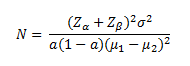In the above equation, µ1- µ2  is the expected difference between two means and σ2 is the common variance of the continuous variable.

Power is lowered as the proportion of the population (a) with an attribute becomes further away from 0.5, which in turn indicates that the proposed sample size will only be sufficient to detect larger effects sizes as the prevalence of the attribute decreases. In general, effect sizes that can be detected in this study are small to moderate (if the whole study size is used) and moderate to large (if a subgroup analysis is performed).

Below we present a graph showing the power of a two-group comparison of a continuous measure as a function of the effect size [(µ1- µ2)/σ] and a common sample size in the two groups.

Comparison of Two Group Means

As a Function of Effect Size and the Sample Size

α = 0.05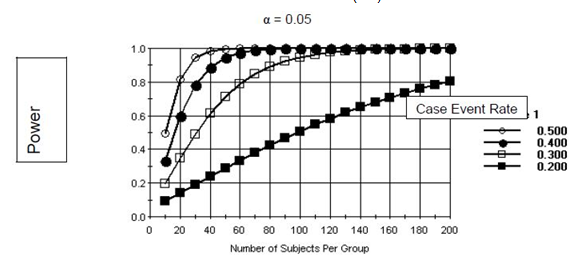As can be seen from the graph, if substantial effect sizes can be hypothesized, the number of required samples for a study will be small. For instance, if the effect size is one half of a standard deviation, a sample size of at least 80 per group will be sufficient to detect this effect when testing at the a= 0.05 level. To have adequate power to detect smaller effects will require larger sample sizes. It is likely that most effects sizes being proposed in the LTRC will fall into the range presented in the above figure.

Analysis of Proportions

Study size calculations for analyses involving proportions are functions of the overall study size, the proportion of participants in each of the comparison groups and the difference between the expected proportion of events in the different groups (9). A study size calculation for a two-group comparison of proportions has the following formula: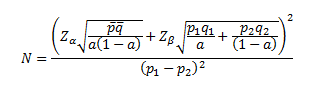In the above equation, p1 is the probability that someone with the characteristic will have a particular event, p2 is the probability that someone without the characteristic will have the event,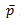is the weighted average (weighted by a) of p1 and p2, and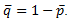.

p~ Studies to compare two groups that include most participants (for example N=1600 and “a” near 0.5) can have low values of "a" (e.g., 0.1) and low probabilities of outcomes (e.g., 0.1) and still have adequate power to detect relative risks of 2 (93%). However, if a subgroup analysis of 300 participants is performed (such as might be the case if two groups were being compared that only differed with respect to the timing of disease progression), both "a" and the probability of and outcome would have to be higher (e.g., "a" = 0.3, probability of an outcome = 0.2, power = 0.94 to detect a relative risk of 2.0).

Below we have presented two figures showing the power to detect specified alternatives of p1 and p2 assuming that equal numbers of specimens are used in each group, and the proportion of specimens with an attribute (the control event rate) is low (0.1) or moderate (0.3). In both of these figures, we have assumed that the proportion of case specimens with the attribute (the case event rate) will be higher in the alternative. As can be seen from these figures, small sample sizes will be sufficient to detect large differences between event rates. If smaller differences between the two event rates are postulated in the alternative hypothesis, larger study sizes will be necessary.

Comparison of Two Proportions

Control Event Rate Low (0.1)Comparison of Two Proportions

Control Event Rate Moderate (0.3)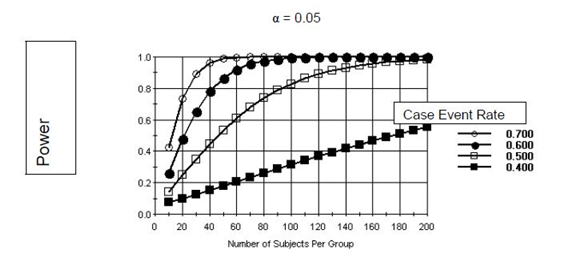Missing Data and Compliance

The DCC will adjust study size requirements using the methods of Lachin (8). Under an assumption that data are missing at random one divides the complete data study size by the estimated proportion of individuals expected to have complete studies to arrive at the final study size estimate.

Regression Models

Multivariate regression models allow one to compare the statistical strength of associations among several risk factors in the presence of markers and co-factors. Power is usually increased when using regression models compared to simple univariate comparisons. For continuous endpoints, inclusion of important independent variables in the regression equation serves to reduce the error variance for all other comparisons. For logistic regression, there is also a bias in estimation of the odds ratio, but the direction of the bias can be positive or negative. Thus, regression models are important because they increase the efficiency of proposed comparisons. However, it is required to insure that there are sufficient numbers of participants to allow regression analyses to take place. LTRC investigators will use the convention of having at least 10 observations for each planned regressor (independent variable) in a multivariate analysis to insure that the sample size is adequate for this type of analysis.

This page is associated with a larger collection of pages. You can view them as a whole here.